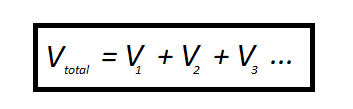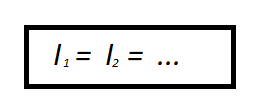# Electric circuits

In this chapter you will learn:

1. What is an electric circuit?

2. What are electric charge and current?

3. What are Potential difference and resistance?

4. Different types of components.

5. What are Series and Parallel circuits?

6. Mains electricity

7. What is National Grid?

8. What are Transformers and their types?

## Electrical charge and current

Charge is a fundamental property of particles. There are two types of charges: positive and negative. A particle with charge experiences a force in an electric field. Electric charge is measured in coulombs, C.

## Current

Electrical current is a flow of electrons (charge) in an electric circuit. The unit of electric current is ampere. An ammeter is used to measure the current in a circuit. A switch is used to turn a circuit on or off.

As per the definition above we can see that electric current and charge are closely related.

The equation for calculating the amount of charge passing through a point in a circuit is:

charge = current × time

The units used in the equation above are as follows:

• charge is measured in coulombs, C

• current is measured in amps, A

• time is measured in seconds, s

Worked examples:

Question 1

A current of 3 amps (A) flows through an electrical circuit.

How much charge will flow past a point in 20 seconds?

charge = current × time

charge = 3 × 20

charge = 60 C

Question 2

How much current flows when a charge of 30 C passes down a wire in 10 seconds?

charge = current × time

Here, we need to change the subject of the equation from charge to current. The rearranged form of the equation is:

current = charge ÷ time

current = 30 ÷ 10

current = 3 A

## Direct and Alternating current

There are two types of current: Direct and Alternating.

• Direct Current (DC) is the flow of electric charge in one direction. Direct current is produced by sources such as batteries and solar cells.

• Alternating Current (AC) is the flow of electric charge that periodically reverses direction. The power provided to our homes is Alternating current.

## Potential difference and resistance

The potential difference is the difference between the amount of energy that charge carriers have at two different points in a circuit. When charge carriers move through a potential difference, energy is transferred due to the electrical work done. Potential difference, therefore, depends on the energy transferred and the amount of charge. Potential difference is also known as voltage. Potential difference is measured in Volts (V).

Definition of one volt: One volt of potential difference exists when one coulomb of charge transfers one joule of energy.

The potential difference can be calculated using the following equation:

potential difference = energy ÷ charge

The units used in the equation above are as follows:

• potential difference is measured in volts, V

• energy is measured in joules, J

• charge is measured in coulombs, C

Worked examples:

Question 3

What is the potential difference between two points if 4 C of charge shifts 8 J?

potential difference = energy ÷ charge

potential difference = 8 ÷ 4

potential difference = 2 V

A voltmeter is used for measuring electrical potential difference between two points in an electric circuit. A voltmeter is placed in parallel with the component across which the potential difference has to be measured.

The current flowing through a component depends on the potential difference across the component and its resistance.

## Electrical resistance

Electrical resistance is a measure of the opposition to current flow in an electrical circuit. It restricts the flow of charge. Resistance is measured in ohms (Ω).

Ohm's law states that, resistance being constant, the current passing through a conductor is directly propotional to the voltage across the conductor. What this means is, for a fixed resister, increasing the voltage results in a propotional increase in current.

According to Ohm's law, potential difference can be calculated using the following equation:

potential difference = current × resistance

The units used in the equation above are as follows:

• potential difference is measured in volts, V

• current is measured in amps, A

• resistance is measured in ohms, Ω

Worked examples:

Question 4

What is the potential difference if a current of 3 A flows through a resistance of 60 Ω?

potential difference = current × resistance

potential difference = 3 × 60

potential difference = 180 V

Question 5

What is the resistance of a component if 16 V causes a current of 4 A through it?

potential difference = current × resistance

resistance = potential difference ÷ current

resistance = 16 ÷ 4

resistance = 4 Ω

## Components

These are some more common components:

## Thermistor

The thermistor is a type of resistor whose resistivity depends on surrounding temperature. A thermistor can be used in thermostats or fire alarms.

Note: At low temperatures, the resistance of a thermistor goes up. In hot conditions, the resistance of a thermistor goes down.

## Switch

A switch is used to turn a circuit on and off.

## Light-dependent resistor (LDR)

LDR is short for light dependant resistor. In a bright light, the LDR's resistance goes down. In darkness, the LDR's resistance goes up.

LDR can be used in a circuit to automatic night lights, burglar detectors. An LDR is a resistor that is dependant on the intensity of light.

## Fixed resistor

A resistor restricts the flow of electrical current. A fixed resistor has a resistance that does not change.

## Variable resistor

Variable resistor is a resistor that increase or decrease the flow of electric current. Variable resistor change resistance from zero to a certain maximum value. A variable resistor is used in some switches and volume controls.

## Series circuits

In a series circuit, different components are connected one after another forming a linear chain of components in a single loop. In a series circuit, because there is just one path for charge to follow, current is the same in all parts of the circuit. In a series circuit, the total potential difference of the power supply is shared between the components.

Note: In a series circuit, if one component is removed or disconnected the circuit is broken and all components stop working.

Potential difference in a series circuit:Current in a series circuit:## Parallel circuits

In a Parallel circuit, each component is in a separate loop. There are different loops so that the current can split up. In a Parallel circuit, all components share the same voltage.Note: In a Parallel circuit, if components in one loop are removed or disconnected it hardly affects the other loops.

In a Parallel circuit, because there are multiple paths (branches) for charge to follow, sum of all branch currents is equal to the total of all the circuit current.

## Power of electrical appliances

The power of an appliance is the energy that it transfers per second. The more the energy transferred in a given time, the higher the power of the device. Power (P) is measured in watts (W). One watt is equal to one joule per second (J/s).

Power depends on current and potenttial difference. The total energy transferred by an electrical appliance depends on the power of the appliance and the amount of time the appliance is switched on for.Where, E is the energy transferred, P is power and t is time

## Mains electricity

Mains electricity supplied to UK homes is produced by power stations and distributed via the National Grid. Mains electricity is supplied as alternating current (A.C.) and used in homes to power electrical appliances. UK mains voltage is 230 Volts. The frequency of A.C. supply is 50 cycles per second, or 50 hertz.

## The three-pin Plugs

In order to use any electrical appliance you need to connect it to the power supply. This is where a three pin plug is used. A plug connects a device to the mains electricity. A power cable consisting of three copper wires connects the device and the three-pin plug. Copper is used in wires because it is a good counductor. The wires are individually coated with plastic.

### Different components inside the plug

• Live wire: This is the wire through which current enters the circuit. The live wire is colour coded in brown. The live wire has a voltage of 230 V.

• Neutral wire: The neutral wire completes the circuit. This wire is colour coded in blue. The neutral wire has a voltage of 0 V.

• Earth wire: The earth wire is connected to the metalic casing of the device. This wire is coated in stripped plastic. The earth wire provides an important saftey feature. If there is a fault this wire provides a path for current to flow from the device to the ground.

• Fuse: A fuse provides protection against the flow of too much current through the circuit. A fuse is a thin wire contained in a glass tube. The thin wire inside the fuse melts if too much current passes through it, which causes the circuit to break. Different fuses are available for use with different amounts of current.

• All three wires in the cable are also protected by an outer layer of plastic insulation.

• A cable grip that holds the cable firmly so that wire do not become loose.

## Earthing

In the event of fault, the earth wire provides an important saftey feature. If there is any type of fault happens and the live wire is loose, then it will touch the case and that will creat a danger. At that time if any one uses the appliance and touch the live metal part, could get an electric shock (electrocuted). The earth wire provides a path for current to flow from the device to the ground instead of passing through a person.

## The National Grid

Mains electricity is distributed via the National Grid. The Natonal Grid connects power stations, where electricity is produced, to homes, where electricity is consumed. National Grid consists of transformers and transmission lines. Electricity demand changes through the day.

## Transformers

A transformer is a device that changes potential difference. A transformer is used to change voltages and currents in transmission lines. Transformers can only work with alternating current.

Note: A transformer is a device that is formed from two coils of wire wound around a magnetic core commonly made of iron.

The number of coils determines wheather the transformerS will step up or step down the voltage. A step-up transformer increases the voltage and a step-down transformer reduces the voltage. A step-up transformer is used to increase the voltage and reduce the current.

Tall metallic towers (Pylons) are used to support transmission lines high above the ground, to keep people safe from these high voltage wires.